Courses

# Anaerobic and Aerobic Treatment Biochemical Kinetics Computer Science Engineering (CSE) Notes | EduRev

## Computer Science Engineering (CSE) : Anaerobic and Aerobic Treatment Biochemical Kinetics Computer Science Engineering (CSE) Notes | EduRev

The document Anaerobic and Aerobic Treatment Biochemical Kinetics Computer Science Engineering (CSE) Notes | EduRev is a part of the Computer Science Engineering (CSE) Course Environmental Engineering - Notes, Videos, MCQs & PPTs.
All you need of Computer Science Engineering (CSE) at this link: Computer Science Engineering (CSE)

BASIC EQUATION FOR BIOLOGICAL TREATMENT OF WASTEWATER

According to Monod kinetics,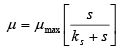(4.2.1)
Where, s is the substrate concentration, ks is the substrate concentration when µ(=µmax/2), µmax is the maximum µ when substrate is not limiting. Also, solid production rate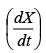is related to substrate utilization rate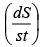by
following relationship: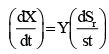(4.2.2)
Where, Sr is the mass of soluble substrate (i.e. BOD), Y is the yield coefficient (kg of new cells formed/kg BOD removed). However owing to large treatment time in many of the large treatment units, substantial number of cells may die because of endogenous respiration. Therefore,

Net production rate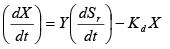(4.2.3)

Where, Kd is the endogenous respiration decay rate constant. For growth phase only,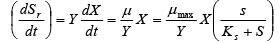(4.2.3)

Case 1- S>>Ks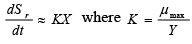(4.2.4)
i.e. removal rate is independent of substrate concentration and that the removal rate depends on X only.

Case 2: S<< KS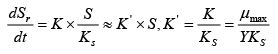(4.2.5)

Here, removal rate depends both upon X and S. Where, X is the mass of biomass in the system (usually represented by MLSS i.e. Mixed Liquor Suspended Solid), µ is the specific growth rate constant (time-1).

Major Terms [a] Hydraulic detention time,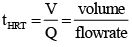(4.2.6)

[b] Sludge age or mean residence time(θC)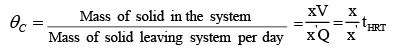(4.2.7)

Where, x (=X/V) the concentration of microbial solution in the system, x’ is the concentration of solids withdrawn.

For the flow through system, x’ = x and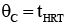For the flow system with recycling, x’ < x and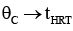[c] Food to microorganism ratio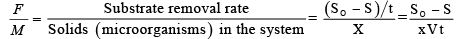(4.2.8)

Problem 4.2.1: An aerated activated sludge tank is being operated under following conditions: Q=4400 m3/d, MLSS=3500 mg/l, Y=0.5, tank volume =770 m3, Endogeneous decay rate constant kd=0.09 d-1.
(a) Estimate weight of solids produced per day for the conditions in which BOD is reduced from 350 mg/l to 130 mg/l.
(b) Estimate θc.
(c) Estimate F/M ratio.

Solution: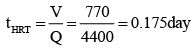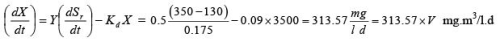=241.4 kg/d.

θc  = mass of solid in the system/ mass of solid leaving the system per day =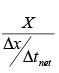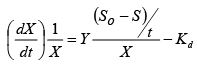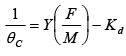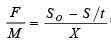= 0.359 (kg BOD/kg MLSS)

θc = 11.17 day.

Offer running on EduRev: Apply code STAYHOME200 to get INR 200 off on our premium plan EduRev Infinity!

,

,

,

,

,

,

,

,

,

,

,

,

,

,

,

,

,

,

,

,

,

;